Marrying the strategic formation models

## Strategic and Random¶

We've got utility from forming subgraphs, links, triangles, etc. But we're going to do is noise that up by putting in some randomness in the utility.

## Example: Social Pressure¶

• Caste relationships
• Are they more likely to occur "in private" with no friends in common
• Or occur with same frequency in embedded relationships?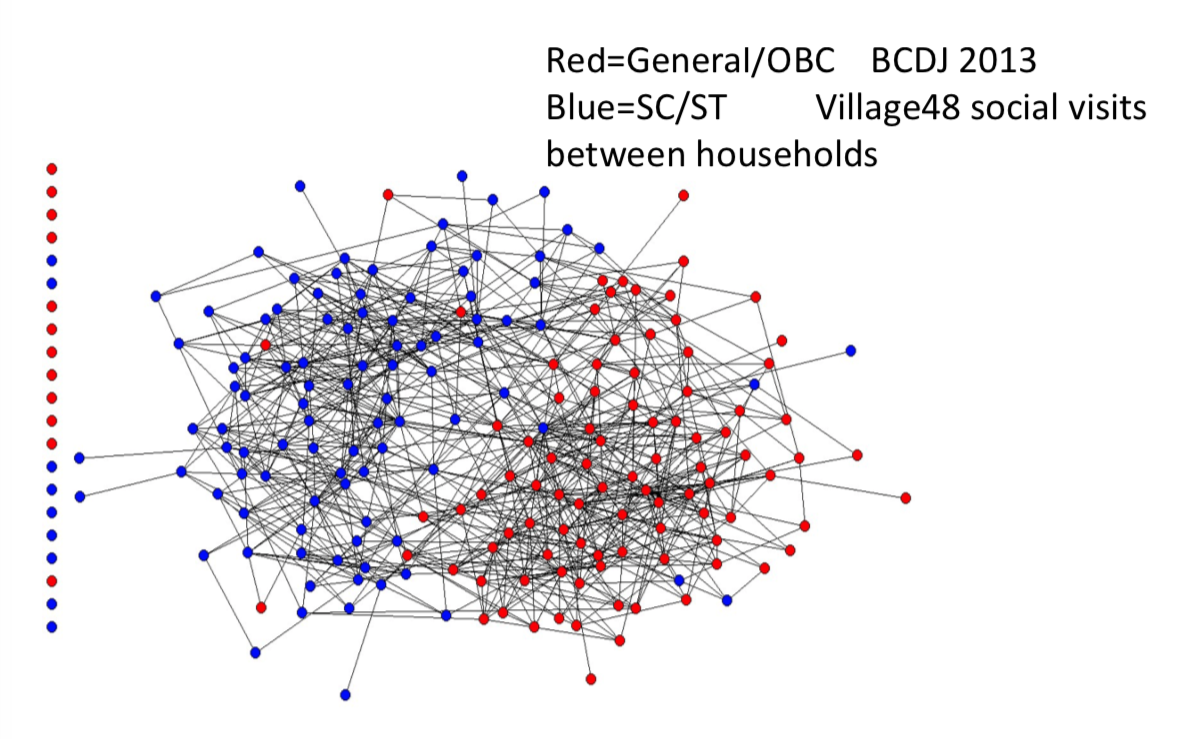## Application : Social Pressure¶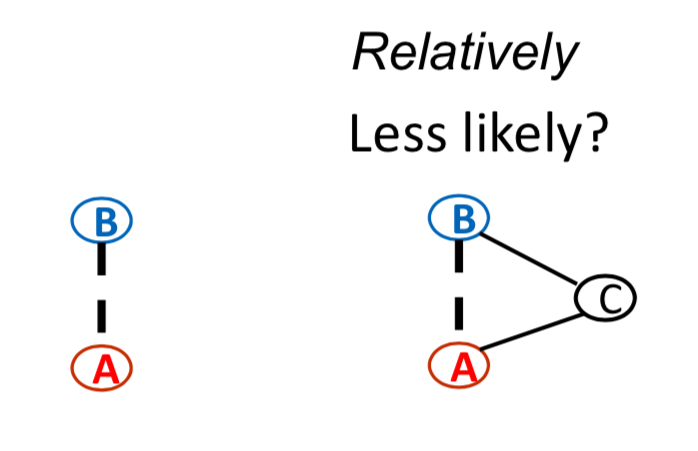## Preferences:¶

Need more consent to form triad than dyad

Need to account for preferences, otherwise will naturally find

            <



## Preference based SUGM:¶

Probability of a link forming depends on likelihood that pair meets, and both wish to form it

• $X_i$: i's characteristics

• $U_L(X_i, X_j) - \epsilon_{ij}$: utility of a link between i and j, based on their characteristics and other person's characteristics, and then something either unobserved or some personality or something else which then also affects that utility, so we'll put it in error terms($\epsilon_{ij}$)

• i benefits from the link iff: $\epsilon_{ij} < U_L(X_i, X_j)$

• pairwise stability: links form if and only if

• $\epsilon_{ij} < U_L(X_i, X_j)$ and $\epsilon_{ji} < U_L(X_j, X_i)$
• $F_L(X_i, X_j)$: distribution of $\epsilon_{ij}$

• prob link forms prop to $F_L(U_L(X_i, X_j))F_L(U_L(X_jX_i))$
• assumption: the errors are iid, so the noise in the chance that j like i isn't dependent with the noise that i get from the same relationship.

## Triangles form¶

Probability of a triangle forming proportional to

$F_T(U_T(X_i, X_j, X_k))F_T(U_T(X_i,X_j,X_k))F_T(U_T(X_k, X_j, X_i))$

## Application: Social Pressure¶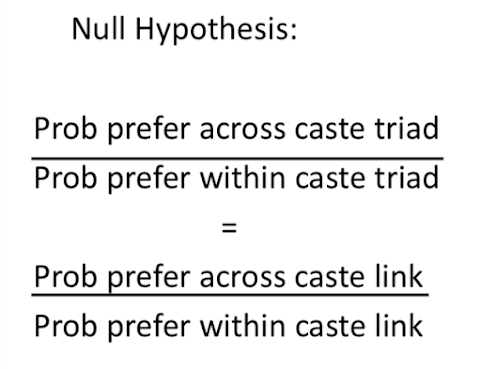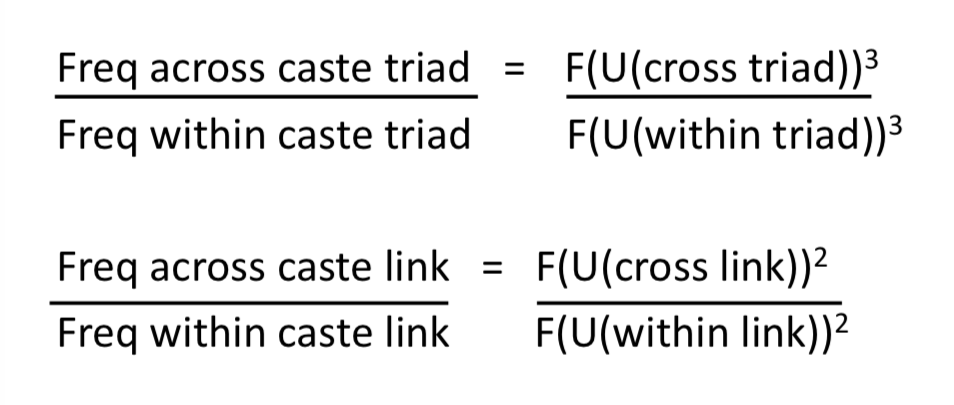#### So¶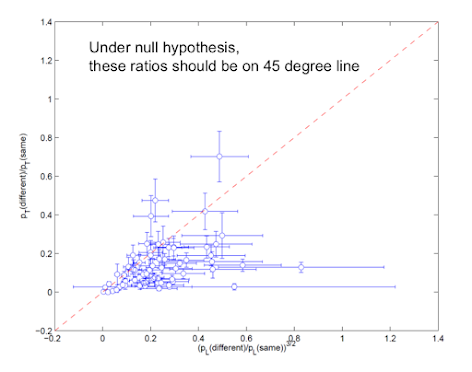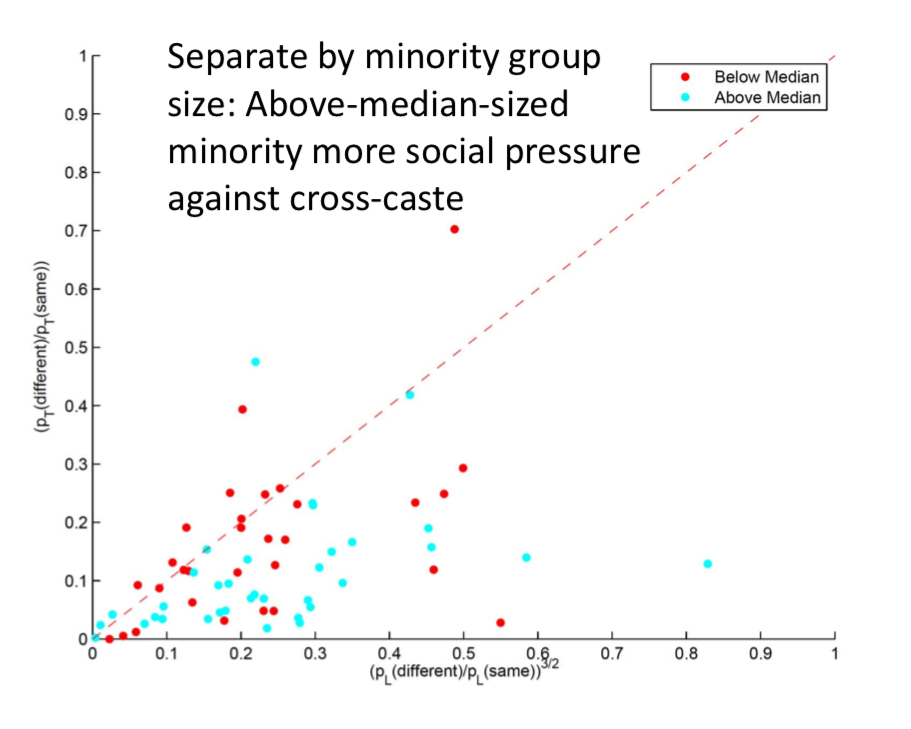## Social Pressure Conclusion¶

• Reject the null hypothesis at the 99.9% level
• Based on model, people show a significantly stronger preference for forming cross-caste relationships when the link is in isolation: no friends in common

## Modeling Strategic Network Formation in a Statistical Model¶

• SUGMs / SERGMs allow for strategic network estimation
• Opens possibilities for systematic estimation
• Subgraphs included, dynamic models...